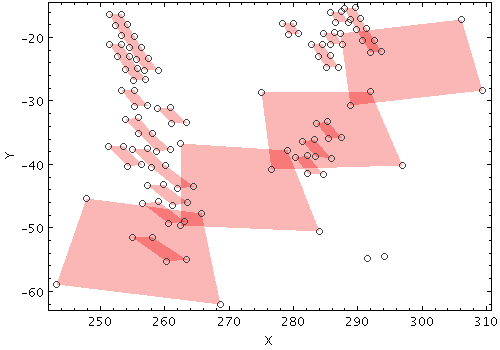Next Previous Up Contents
Next: polygon
Up: Layer Types
Previous: poly4

#### 8.3.11 `mark4`

Plots 4 similar markers of fixed size and shape representing 4 separate positions from the same input table row. This is a convenience option that can be used with other plot layers based on 4 positions.

Usage Overview:

```   layerN=mark4 shapeN=filled_circle|open_circle|... sizeN=<pixels>
<pos-coord-params1N> <pos-coord-params2N> <pos-coord-params3N>
<pos-coord-params4N> inN=<table> ifmtN=<in-format>
istreamN=true|false icmdN=<cmds>
```

All the parameters listed here affect only the relevant layer, identified by the suffix `N`.

Positional Coordinate Parameters:
The positional coordinates `<pos-coord-params1N>` , `<pos-coord-params2N>` , `<pos-coord-params3N>` , `<pos-coord-params4N>` give 4 positions for each row of the input table. Their form depends on the plot geometry, i.e. which plotting command is used. For a plane plot (`plot2plane`) the parameters would be `x1N`, `y1N`, `x2N`, `y2N`, `x3N`, `y3N`, `x4N` and `y4N`. The coordinate parameter values are in all cases strings interpreted as numeric expressions based on column names. These can be column names, fixed values or algebraic expressions as described in Section 10.

Example:```   stilts plot2plane in=big_tab_VIR_VIS_CSA_public.fits
icmd='select IOF_055<0.005'
icmd='select lon_center>250&&lon_center<300&&lat_center>-65&&lat_center<-16'
x1=LON_CORNER_1 y1=LAT_CORNER_1
x2=LON_CORNER_2 y2=LAT_CORNER_2
x3=LON_CORNER_3 y3=LAT_CORNER_3
x4=LON_CORNER_4 y4=LAT_CORNER_4
layer_m=mark4 color_m=404040 shape_m=open_circle size_m=3
```

`icmdN = <cmds>`       (ProcessingStep[])
Specifies processing to be performed on the layer N input table as specified by parameter `inN`. The value of this parameter is one or more of the filter commands described in Section 6.1. If more than one is given, they must be separated by semicolon characters (";"). This parameter can be repeated multiple times on the same command line to build up a list of processing steps. The sequence of commands given in this way defines the processing pipeline which is performed on the table.

Commands may alteratively be supplied in an external file, by using the indirection character '@'. Thus a value of "`@filename`" causes the file `filename` to be read for a list of filter commands to execute. The commands in the file may be separated by newline characters and/or semicolons, and lines which are blank or which start with a '`#`' character are ignored.

`ifmtN = <in-format>`       (String)
Specifies the format of the input table as specified by parameter `inN`. The known formats are listed in Section 5.1.1. This flag can be used if you know what format your table is in. If it has the special value `(auto)` (the default), then an attempt will be made to detect the format of the table automatically. This cannot always be done correctly however, in which case the program will exit with an error explaining which formats were attempted. This parameter is ignored for scheme-specified tables.

[Default: `(auto)`]

`inN = <table>`       (StarTable)
The location of the input table. This may take one of the following forms:
• A filename.
• A URL.
• The special value "`-`", meaning standard input. In this case the input format must be given explicitly using the `ifmtN` parameter. Note that not all formats can be streamed in this way.
• A scheme specification of the form `:<scheme-name>:<scheme-args>`.
• A system command line with either a "`<`" character at the start, or a "`|`" character at the end ("`<syscmd`" or "`syscmd|`"). This executes the given pipeline and reads from its standard output. This will probably only work on unix-like systems.
In any case, compressed data in one of the supported compression formats (gzip, Unix compress or bzip2) will be decompressed transparently.
`istreamN = true|false`       (Boolean)
If set true, the input table specified by the `inN` parameter will be read as a stream. It is necessary to give the `ifmtN` parameter in this case. Depending on the required operations and processing mode, this may cause the read to fail (sometimes it is necessary to read the table more than once). It is not normally necessary to set this flag; in most cases the data will be streamed automatically if that is the best thing to do. However it can sometimes result in less resource usage when processing large files in certain formats (such as VOTable). This parameter is ignored for scheme-specified tables.

[Default: `false`]

`shadingN = auto|flat|translucent|transparent|density|aux|weighted <shade-paramsN>`       (ShapeMode)
Determines how plotted objects in layer N are coloured. This may be influenced by how many objects are plotted over each other as well as the values of other parameters. Available options (Section 8.4) are:
• `auto`
• `flat`
• `translucent`
• `transparent`
• `density`
• `aux`
• `weighted`
Each of these options comes with its own set of parameters to specify the details of how colouring is done.

[Default: `auto`]

`shapeN = filled_circle|open_circle|...`       (MarkerShape)
Sets the shape of markers that are plotted at each position of the scatter plot.

The available options are:

• `filled_circle`
• `open_circle`
• `cross`
• `x`
• `open_square`
• `open_diamond`
• `open_triangle_up`
• `open_triangle_down`
• `fat_circle`
• `fat_cross`
• `fat_x`
• `fat_square`
• `fat_diamond`
• `fat_triangle_up`
• `fat_triangle_up`
• `filled_square`
• `filled_diamond`
• `filled_triangle_up`
• `filled_triangle_down`

[Default: `filled_circle`]

`sizeN = <pixels>`       (Integer)
Size of the scatter plot markers. The unit is pixels, in most cases the marker is approximately twice the size of the supplied value.

[Default: `1`]

Next Previous Up Contents
Next: polygon
Up: Layer Types
Previous: poly4

STILTS - Starlink Tables Infrastructure Library Tool Set